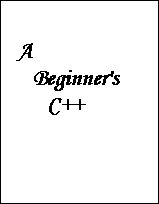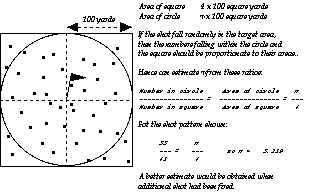This page contains text from the draft version of a book "A Beginners' C++"; this text is intended for "CS1, CS2" introductory Computer Science courses that use C++ as an implementation language.

This particular page contains one of the examples from the chapter that introduces "functions". The example makes use of the random number generator rand().

# 10: Functions

### 10.11.1 Pi-Canon

A mathematics professor once approached a colonel of his state's National Guard asking for the Guards assistance in a new approach to estimating the value of Pi.

The professor reckoned that if a cannon was fired at a distant target flag, the balls would fall in a random pattern around the target. After a large number of balls had been fired, he would be able to get an estimate of Pi by noting where the balls had fallen. He used the diagram shown in Figure 10.3, to explain his approach to estimating Pi:

The colonel refused. He reckoned that his gunners would put every shot within 50 paces of the target flag and that this would lead to the result Pi = 4 which everyone knew was wrong.

Write a computer program that will allow the maths. professor to simulate his experiment.

The program should let the professor specify the number of shots fired. It should simulate the experiment printing estimates of Pi at regular intervals along with a final estimate when all ammunition has been expended.#### Design

The design process appropriate to problems of this complexity is known as "top-down functional decomposition". We know the overall function (the "top function" of the program), it is to run the simulation and calculate Pi. But obviously, that involves a lot of work. It is far too much work to be encoded in a single main function. So, we proceed by iterating through the problem repeatedly, at each iteration we try to refine our model and abstract out distinct subproblems that can be analyzed separately.

The problem obviously involves random numbers. For each "shot" we will need two random numbers, one to give the "x" position relative to the flag, the second to give the "y" position. We can arrange it so that all shots fall in the square by selecting the range for the random numbers (just have to tell the professor that we are ignoring bad shots that fall too far from the target). When we have the x, y coordinates of where the shot is supposed to have landed, we can calculate the distance from the flag. If this distance is less than 100.0, the shot adds to the count of those falling in the circle. We can get an estimate of Pi whenever we need by just doing the calculation using our counts of total shots fired and shots landing in the circle.

##### First iteration through design

Based on the problem description, we can identify a rough framework for the program:

```//initialization phase
get professor to say how much ammunition he has
get some seed for the random number generator
loop until ammunition exhausted
increment count of shots fired
get x, y coordinates
test position and, if appropriate,
update count of shots in circle
maybe print next estimate of Pi (not for every shot,
What is frequency of these reports?)
print final estimate of Pi
```
Some "functions" appear immediately in this description, for example, "estimate Pi", "test position", "get coordinate". All of these tasks involve several calculation steps that clearly belong together, can be thought about in isolation, and should be therefore separate functions. Even something like "initialization phase" could probably be a function because it groups related operations.
##### Second iteration through design

We should now start to identify some of these functions:

```get ammunition
prompts professor for inputs like amount of ammunition,
and seed for random number generator
use seed to seed the random number generator
return amount of ammunition

Pi-estimator function
must be given total shot count and circle shot count
calculates Pi from professor's formula
returns Pi estimate

get-coordinate function
use random number generator, converts 0..32767 result
into a value in range -100.0 to +100.0 inclusive
returns calculated value

print Pi-estimate
produce some reasonably formatted output,
probably should show Pi and the number of shots used to
get this estimate, so both these values will
be needed as arguments

test in circle
given an x and y coordinate pair test whether they
represent a point within 100.0 of origin
return 0 (false) 1 (true) result

main function
get ammunition
loop until ammunition exhausted (How checked?)
increment count of shots fired
(Where did this get initialized?)
x = get-coordinate
y = get-coordinateget x,y coordinates
if test in circle (x, y)
increment circle count
maybe print next estimate of Pi (not for every shot,
What is frequency of these reports?)
call print Pi estimate

```
There are some queries. These represent issues that have to be resolved as we proceed into more detailed design.
##### Third iteration through design process

This problem is still fairly simple so we have already reached the point where we can start thinking about coding. At this point, we compose function prototypes for each of the functions. These identify the data values that must be given to the function and the type of any result:

##### Composing the function prototypes
```long	GetAmmunition(void);

double	PiEstimate(long squareshot, long circleshot);

double	GetCoordinate(void);

void	PrintPIEstimate(double pi, long shotsused);

int		TestInCircle(double x, double y);

int		main();
```

#### Final stage design and implementation

Next, we have to consider the design of each individual function. The process here is identical to that in Part II where we designed the implementations of the little programs. We have to expand out our english text outline for the function into a sequence of variable definitions, assignment statements, loop statements, selection statements etc.

Most of the functions that we need here are straightforward:

```long GetAmmunition(void)
{
long	munition;
long	seed;
cout << "Please Professor, how many shots should we fire?";
// Read shots, maybe we should use that GetIntegerInRange
// to make certain that the prof enters a sensible value
// Risk it, hope he can type a number
cin >> munition;
// Need seed for random number generator,
// Get prof to enter it (that way he has chance of
// getting same results twice).  Don't confuse him
// with random number stuff, just get a number.
cout << "Please Professor, which gunner should lay the gun?"
<< endl;
cout << "Please enter a gunner identifier number 1..1000 :";
cin >> seed;
srand(seed);
return munition;
}

int TestInCircle(double x, double y)
{
// Use the sqrt() function from math.h
// rather than NewtRoot()!
// Remember to #include
double distance = sqrt(x*x + y*y);
return distance < 100.0;
}

double PiEstimate(long squareshot, long circleshot)
{
return 4.0*double(circleshot)/squareshot;
}
```

The GetCoordinate() function is slightly trickier; we want a range -100.0 to +100.0. The following should suffice:

```double GetCoordinate(void)
{
int	n = rand();
// Take n modulo 201, i.e. remainder on dividing by 201
// That should be a number in range 0 to 200
// deduct 100
n = n % 201;
return double(n-100);
}
```

The main() function is where we have to consider what data values are needed for the program as a whole. We appear to need the following data elements:

```long counter for total shots
long counter for shots in circle
long counter for available munitions

double for Pi estimate
```
within the loop part of main() we also require:
```double x coordinate
double y coordinate
```
We have to decide how often to generate intermediate printouts of Pi estimates. If professor is firing 1000 shots, he would probably like a report after every 100; if he can only afford to pay for 100 shots, he would probably like a report after every 10. So it seems we can base report frequency on total of shots to be fired. We will need a couple of variables to record this information; one to say frequency, the other to note how many shots fired since last report. So, main() will need two more variables.
```long counter for report frequency
long counter for shots since last report
```
We can now complete a draft for main():
```int main()
{
long	fired = 0, ontarget = 0, ammunition;
double	pi = 4.0; // Use the colonel's estimate!
long	freq, count;

ammunition = GetAmmunition();

freq = ammunition / 10;
count = 0;

for(; ammunition > 0; ammunition--) {
double x, y;
fired++;
x = GetCoordinate();
y = GetCoordinate();
if(TestInCircle(x, y))
ontarget++;

count++;
if(count == freq) {
// Time for another estimate
pi = PiEstimate(fired, ontarget);
PrintPIEstimate(pi, fired);
// reset count to start over
count = 0;
}
}

cout << "We've used all the ammunition." << endl;
cout << "Our final result:" << endl;
pi = PiEstimate(fired, ontarget);
PrintPIEstimate(pi, fired);
return 0;
}
```

Complete the implementation of the program (you need to provide PrintPIEstimate()) and run it on your system.

The results I got suggest that the prof. would have to apply for, and win, a major research grant before he could afford sufficient ammunition to get an accurate result:

```Estimate for pi, based on results of 1000 shots, is 3.24800000
Estimate for pi, based on results of 10000 shots, is 3.12160000
```